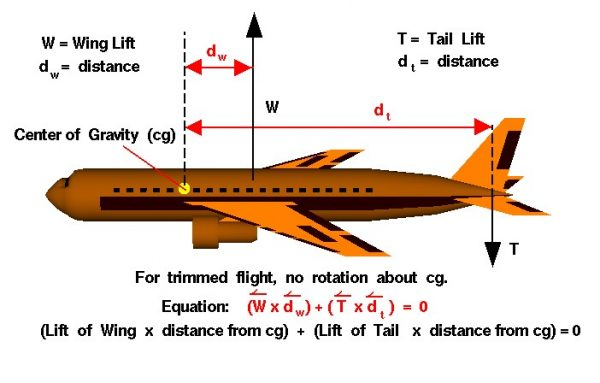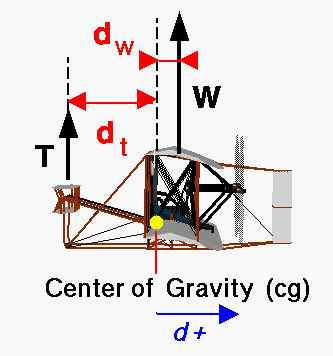# Trimmed Aircraft## Lift

As described on the forces slide, the aircraft lift is the sum of the lift of all of the parts of the airplane and acts through the aircraft center of pressure. Each part of the aircraft has its own lift component and its own center of pressure. The major part of the lift comes from the wings, but the horizontal stabilizer and elevator also produce lift which can be varied to maneuver the aircraft.

## Center of Gravity (cg)

The average location of the weight of the aircraft is the center of gravity (cg). Any force acting at some distance from the cg produces a torque about the cg. Torque is defined to be the product of the force times the distance. A torque is a “twisting force” that produces rotations of an object. In flight, during maneuvers, an airplane rotates about its cg. But when the aircraft is not maneuvering, we want the rotation about the cg to be zero. When there is no rotation about the cg the aircraft is said to be trimmed.

On most aircraft, the center of gravity of the airplane is located near the center of pressure of the wing. If the center of pressure of the wing is aft of the center of gravity, its lift produces a counter-clockwise rotation about the cg. The center of pressure for the elevator is aft of the center of gravity for the airliner shown in the figure. A positive lift force from the tail produces a counter-clockwise rotation about the cg. To trim the aircraft it is necessary to balance the torques produced by the wing and the tail. But since both rotations are counter-clockwise, it is impossible to balance the two rotations to produce no rotation. However, if the tail lift is negative it then produces a clockwise rotation about the cg which can balance the wing rotation.

## Torque

Let us look carefully at the torques produced by the wing and the tail. The torque from the wing TW is equal to the lift of the wing W times the distance from the cg to the center of pressure of the wing dw.

### TW = W * dw

The torque from the tail TT is equal to the lift of the tail T times the distance from the cg to the center of pressure of the tail dt. The lift of the wing and the lift of the tail are both forces and forces are vector quantities which have both a magnitude and a direction. We must include a minus sign on the lift of the tail because the direction of this force is negative.

### TT = -T * dt

In trimmed flight, these two torques are equal:

## Vector Quantities

The torque equation, as written here, is a vector equation. All of the quantities are vector quantities having a magnitude and a direction. If the distances are both positive (same side of the center of gravity), then the direction of the tail force must be different than the direction of the wing force to produce no net torque or rotation. However, if the distance to the tail were negative, then the lift of the of the tail could be positive and there would be no net torque. A negative distance to the tail would imply that the tail is on the front of the aircraft, ahead of the center of gravity. A tail at the front of the aircraft is called a canard and was the configuration first used by the Wright brothers.## Vector Sum

The total lift of the aircraft is the vector sum of the wing lift and the tail lift. For the airliner, the total lift is less than the wing lift; for the Wright brothers, the total lift is greater than the wing lift. The added lift was important for the Wright brothers because their aircraft had a very small engine and flew at low speeds (35mph). Since lift depends on the square of the velocity, it is hard to generate enough lift for flight at such low speeds.

Provide feedback